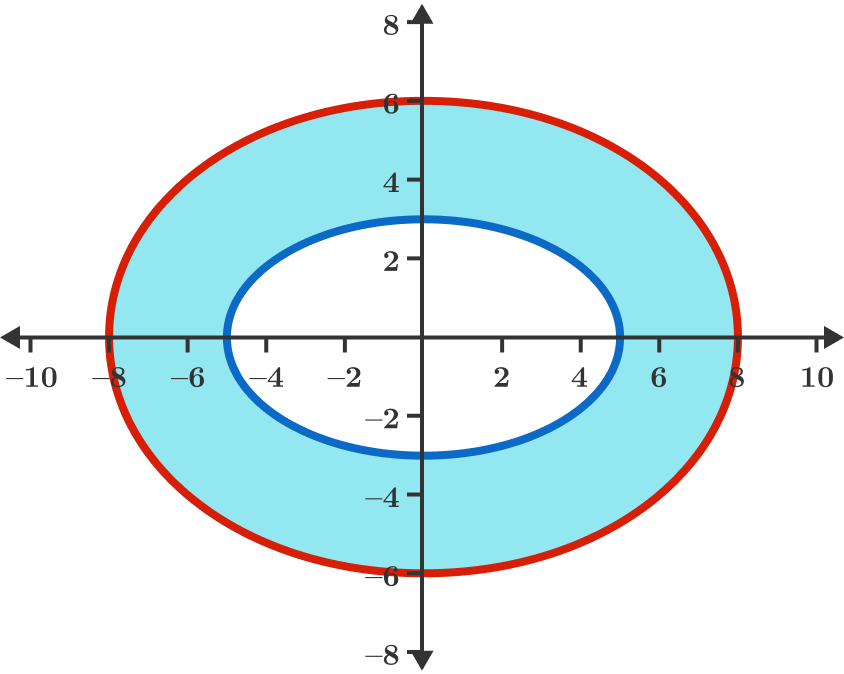# An interesting Curve

Geometry Level 3

Given an ellipse (in blue) with a horizontal major axis of length 10 and a vertical minor axis of length 6, we construct a curve (in red) which is the boundary of the convex hull of points which are 3 units away from the blue ellipse.

Find the length of this red curve, and find the area of the band with width 3 (shaded in light blue) around the ellipse.

If $S$ is the sum of these two numbers, then submit $\lfloor 1000 S \rfloor$ as your answer.×